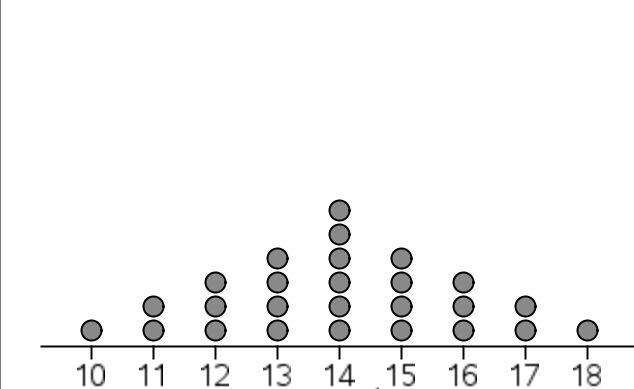# 2116 Univariate Data - DOT Plots

10 Questions | Total Attempts: 408Settings• 1.
Dot plots are used for :
• A.

All categorical data

• B.

All numerical data

• C.

Small numerical data sets

• D.

Small categorical data sets

• E.

Data sets greater than 50

• F.

I don't know

• 2.
When we describe a dot plot, sometimes we :
• A.

Have too little data to comment on the shape of the distribution

• B.

Have too much data to comment on the centre of the distribution

• C.

Have too much to comment on the distribution at all

• D.

Make assumptions about the centre of the distribution

• E.

Add more dots to predict the pattern

• F.

I don't know

• 3.
By simply looking at a dot plot, it is easy to find the
• A.

Modal class

• B.

Standard deviation

• C.

Interquartile range

• D.

Z scores

• E.

Mean

• F.

I don't know

• 4.
When creating a dot plot, when several points share the same value, we :
• A.

Ignore the duplicate value

• B.

Put the value beside the previous dot

• C.

Stack the dots on top of one another

• D.

Change the colour of the dot

• E.

Count the extra dots as outliers

• F.

I don't know

• 5.
The dot plot shown above could be described as :
• A.

Pointy

• B.

Normally distributed

• C.

Symmetrically skewed

• D.

Negatively skewed

• E.

Optimal

• F.

I don't know

• 6.
The dot plot shown above :
• A.

Is negatively skewed

• B.

Is normally distributed

• C.

Symmetrically skewed

• D.

Shows no real shape in its distribution

• E.

Optimal

• F.

I don't know

• 7.
The dot plot shown above :
• A.

Is negatively skewed

• B.

Is normally distributed

• C.

Symmetrically skewed

• D.

Positively skewed

• E.

Optimal

• F.

I don't know

• 8.
The dot plot shown above has a modal class of :
• A.

10

• B.

19

• C.

11

• D.

16

• E.

Less than 12

• F.

I don't know

• 9.
The dot plot shown above :
• A.

Is negatively skewed

• B.

Has a mode of 5

• C.

Is symmetrically skewed

• D.

Shows no real shape in its distribution

• E.

Is optimal

• F.

I don't know

• 10.
The dot plot shown above has a range of :
• A.

10

• B.

19

• C.

11

• D.

16

• E.

9

• F.

I don't knowBack to top